Courses

# Application Of Schrodinger Wave Equation – MSQ

## 10 Questions MCQ Test Topic wise Tests for IIT JAM Physics | Application Of Schrodinger Wave Equation – MSQ

Description
This mock test of Application Of Schrodinger Wave Equation – MSQ for Physics helps you for every Physics entrance exam. This contains 10 Multiple Choice Questions for Physics Application Of Schrodinger Wave Equation – MSQ (mcq) to study with solutions a complete question bank. The solved questions answers in this Application Of Schrodinger Wave Equation – MSQ quiz give you a good mix of easy questions and tough questions. Physics students definitely take this Application Of Schrodinger Wave Equation – MSQ exercise for a better result in the exam. You can find other Application Of Schrodinger Wave Equation – MSQ extra questions, long questions & short questions for Physics on EduRev as well by searching above.
*Multiple options can be correct
QUESTION: 1

### Consider two cases of one dimensional potential well. The first case is an infinite potential will  V = 0,  for  a < x < 0  the second case is a finite potential well. Which of the following is true? [for  E < V0]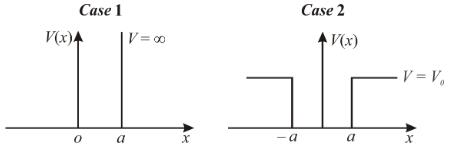Solution:

The correct answers are: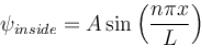for infinite case &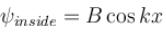for finite case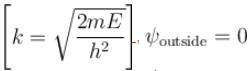for infinite case &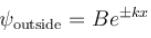for the finite case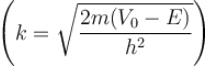*Multiple options can be correct
QUESTION: 2

### If a particle is confined to a one dimensional box of length a  with the centre of the box at x = 0. Then, choose the correct option.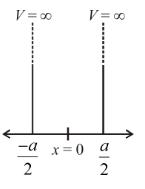Solution:

The case of a symmetric one dimensional box, is similar to the box from x = 0 to a. The wave functions can be derived from the later case by putting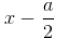in place of x.

The correct answers are: The solutions of this case can be obtained by substituting x  by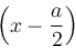in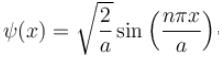The normalization constant will be same as in the case of a box centered x = a/2 , The energy levels are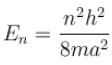*Multiple options can be correct
QUESTION: 3

### For a wave function ψ(x) , its time dependence can be seen from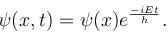Select the correct option.

Solution: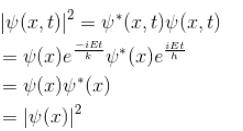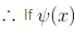is normalised, so is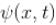& neither of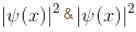has any time dependence.

The correct answers are: If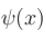is normalised, then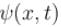has to be normalised, Neither of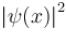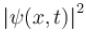has any time dependence

*Multiple options can be correct
QUESTION: 4

Consider the solutions to particle in one dimensional box of length, L.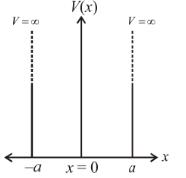Which of the following is true for the group of functions  ψ2(x)

Solution: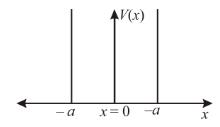The solution of a symmetric infinite potential well consists of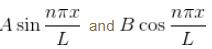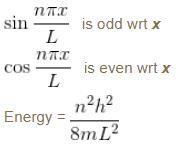Now, any function can be written as a linear combination of these functions (sin and cos)

They form a complete let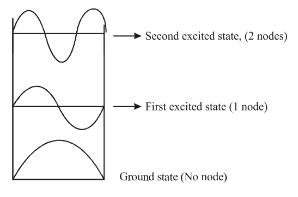The correct answers are: They are alternately even and odd with respect to the centre of the well, As we go up in energy, each successive state has one more node. i.e.  ψ1(x) has none, ψ2(x) has one, ψ3(x) has 2 and so on, They are mutually orthogonal, They form a complete set

*Multiple options can be correct
QUESTION: 5

Choose the correct option.
For the case of particle in a one dimensional box.

Solution: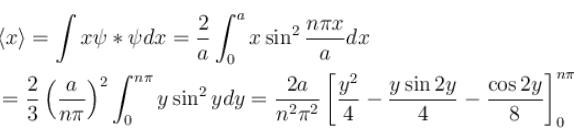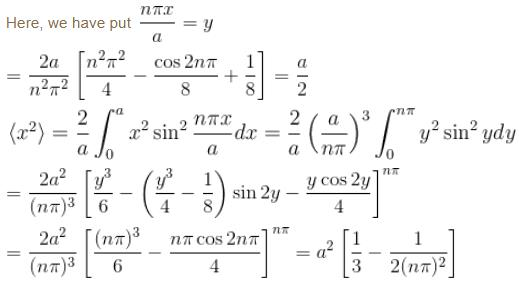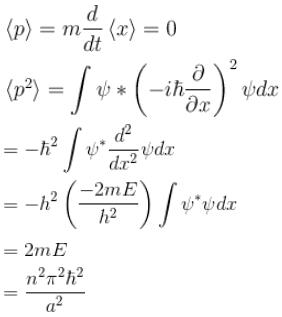The correct answers are: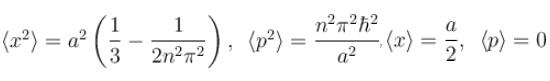*Multiple options can be correct
QUESTION: 6

For the above cases of one-dimensional infinite and finite potential wells which of the following is true?

Solution:

Energy levels are same!

The correct answer is: Energy levels are same,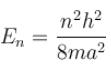in both cases.

*Multiple options can be correct
QUESTION: 7

For a particle in a one dimensional box,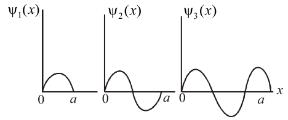There is zero probability of finding the particle at

Solution: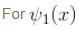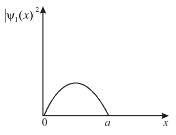Probability is 0 at x = 0 & a
For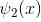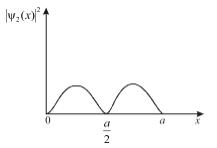Probability density is zero at x = 0, a/2 , a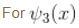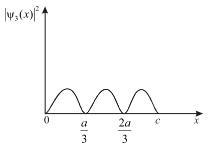Probability density zero at x = 0,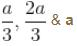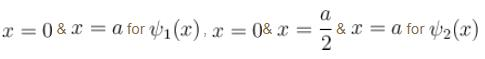*Multiple options can be correct
QUESTION: 8

Which of the following is true in case of a free particle?

Solution:

The Schrodinger equation solution are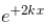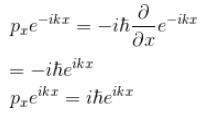∴  momentum eigenfunction.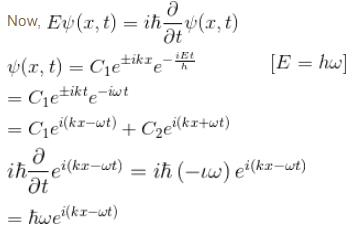The correct answers are: The Schrodinger equation yields the solution to be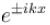(linear combination of the two), The solutions of the Schrodinger equation are both energy and momentum eigenfunctions

*Multiple options can be correct
QUESTION: 9

In any arbitrary potential, an acceptable solution of the Schrodinger equations must satisfy which of the following properties?

Solution:

The correct answers are: The gradient of the solution must be single valued, finite and continuous at the every point, The gradient should also be bounded at large distances, The wave function must be continuous throughout, The wave function must vanish at the point where the potential approaches infinity

*Multiple options can be correct
QUESTION: 10

Which of the following is true for a quantum harmonic oscillator?

Solution: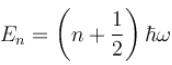for a harmonic oscillator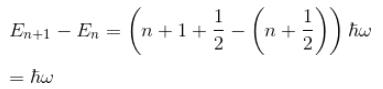∴   evenly spaced.

The correct answers are: A spectrum of evenly spaced energy levels, A non zero probability of finding the oscillator outside the classical turning points